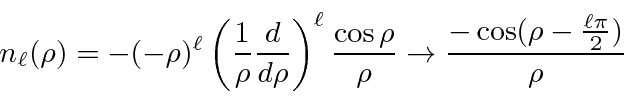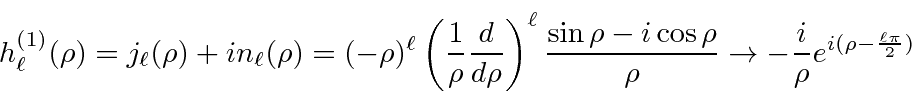## Solutions to the Radial Equation for Constant Potentials

Solutions to the radial equation in a constant potential are important since they are the solutions for largein potentials of limitted range. They are therefore used in scattering problems as the incoming and outgoing states. The solutions are the spherical Bessel and spherical Neumann functions.where. The linear combination of these which falls off properly at largeis called the Hankel function of the first type.We use these solutions to do a partial wave analysis of scattering, solve for bound states of a spherical potential well, solve for bound states of an infinite spherical well (a spherical box''), and solve for scattering from a spherical potential well.

Jim Branson 2013-04-22